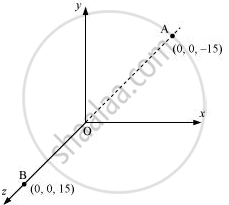# A system has two charges qA = 2.5 × 10−7 C and qB = −2.5 × 10−7 C located at points A: (0, 0, − 15 cm) and B: (0, 0, + 15 cm), respectively. - Physics

Numerical

A system has two charges qA = 2.5 × 10−7 C and qB = −2.5 × 10−7 C located at points A: (0, 0, − 15 cm) and B: (0, 0, + 15 cm), respectively. What are the total charge and electric dipole moment of the system?

#### Solution

Both the charges can be located in a coordinate frame of reference as shown in the given figure.At A, amount of charge, qA = 2.5 × 107C

At B, amount of charge, qB = −2.5 × 10−7 C

Total charge of the system,

q = qA + qB

= 2.5 × 107 C − 2.5 × 10−7 C

= 0

Distance between two charges at points A and B,

d = 15 + 15 = 30 cm = 0.3 m

Electric dipole moment of the system is given by,

p = qA × d = qB × d

= 2.5 × 10−7 × 0.3

= 7.5 × 10−8 C m along positive z-axis

Therefore, the electric dipole moment of the system is 7.5 × 10−8 C m along positive z−axis.

Concept: Electric Dipole
Is there an error in this question or solution?

#### APPEARS IN

NCERT Physics Part 1 and 2 Class 12
Chapter 1 Electric Charges and Fields
Exercise | Q 1.9 | Page 46
NCERT Class 12 Physics Textbook
Chapter 1 Electric Charge and Fields
Exercise | Q 9 | Page 46

Share